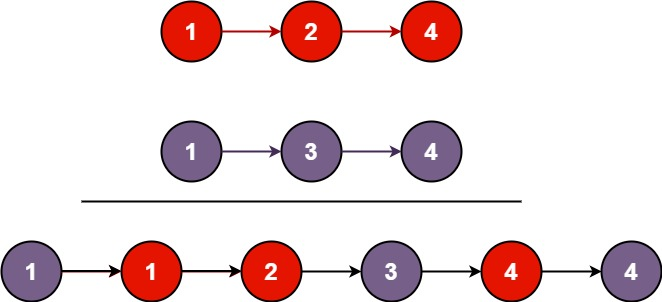# 21. Merge Two Sorted Lists

Updated: 2023-05-04

LeetCode problem

You are given the heads of two sorted linked lists list1 and list2.

Merge the two lists in a one sorted list. The list should be made by splicing together the nodes of the first two lists.

Example 1:Input: list1 = [1,2,4], list2 = [1,3,4]
Output: [1,1,2,3,4,4]


Example 2:

Input: list1 = [], list2 = 
Output: 


## First accepted

Idea:

Get smallest head. Loop and update its next.

# Definition for singly-linked list.
# class ListNode:
#     def __init__(self, val=0, next=None):
#         self.val = val
#         self.next = next
class Solution:
def mergeTwoLists(self, l1: Optional[ListNode], l2: Optional[ListNode]) -> Optional[ListNode]:
res = ListNode()
current = res
while l1 and l2:
if l1.val <= l2.val:
node = ListNode(l1.val)
l1 = l1.next
else:
node = ListNode(l2.val)
l2 = l2.next
current.next = node
current = current.next

if l1:
current.next = l1
if l2:
current.next = l2

return res.next


## Better solution

Recursion

def mergeTwoLists(self, l1: Optional[ListNode], l2: Optional[ListNode]) -> Optional[ListNode]:
if l1 and l2:
if l1.val > l2.val:
l1, l2 = l2, l1 #swap smaller and larger: make l1 the one with the smaller first value
l1.next = self.mergeTwoLists(l1.next, l2) # move forward in the list which starts with the smaller value
return l1 or l2 # return whichever of the two lists remains at the end


Loop

def mergeTwoLists(self, l1: Optional[ListNode], l2: Optional[ListNode]) -> Optional[ListNode]:
res = ListNode()
current = res
while l1 and l2:
if l1.val <= l2.val:
current.next = l1
l1 = l1.next
else:
current.next = l2
l2 = l2.next
current = current.next


class Solution:
def mergeTwoLists(self, a, b):
if a and b:
if a.val > b.val:
a, b = b, a
a.next = self.mergeTwoLists(a.next, b)
return a or b


First make sure that a is the “better” one (meaning b is None or has larger/equal value). Then merge the remainders behind a.

def mergeTwoLists(self, a, b):
if not a or b and a.val > b.val:
a, b = b, a
if a:
a.next = self.mergeTwoLists(a.next, b)
return a